Ex 3.3

Chapter 3 Class 10 Pair of Linear Equations in Two Variables
Serial order wise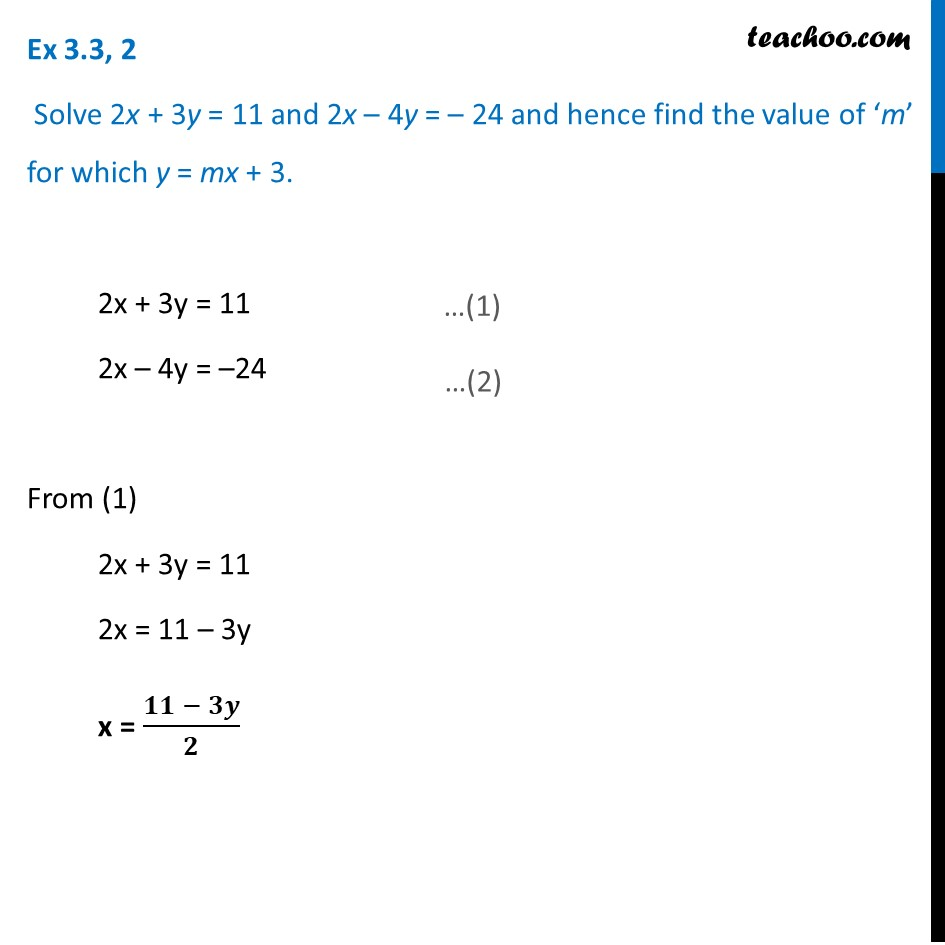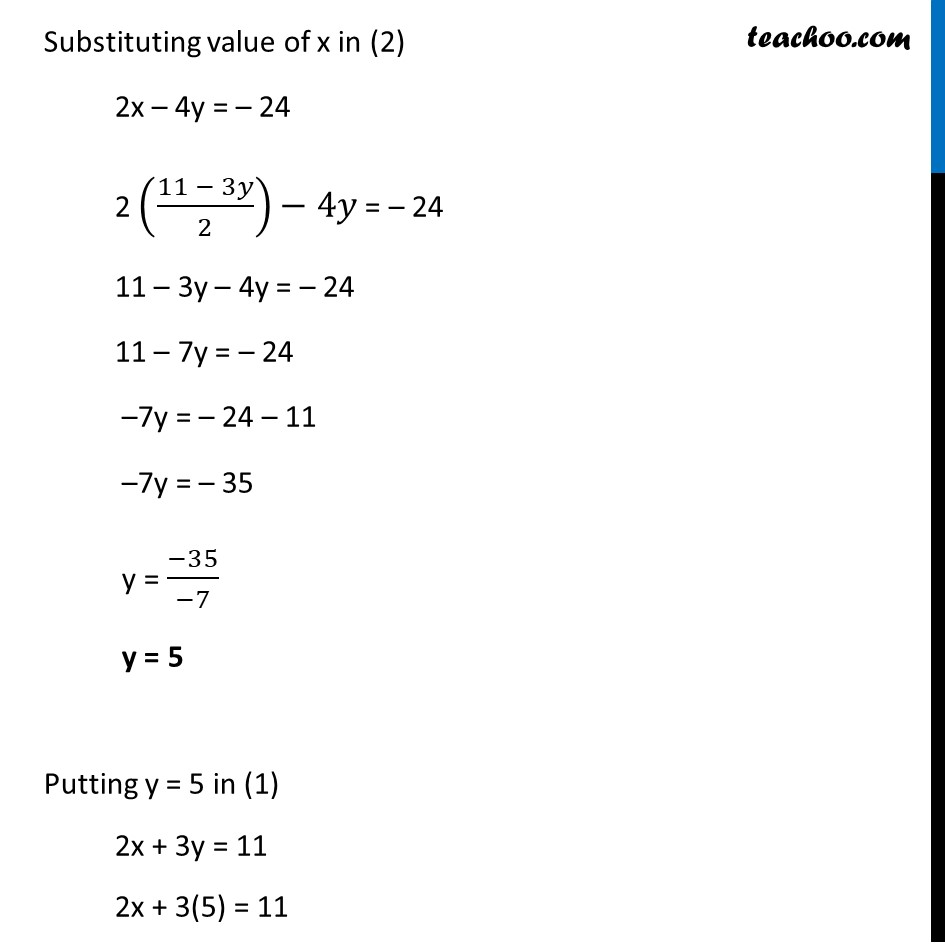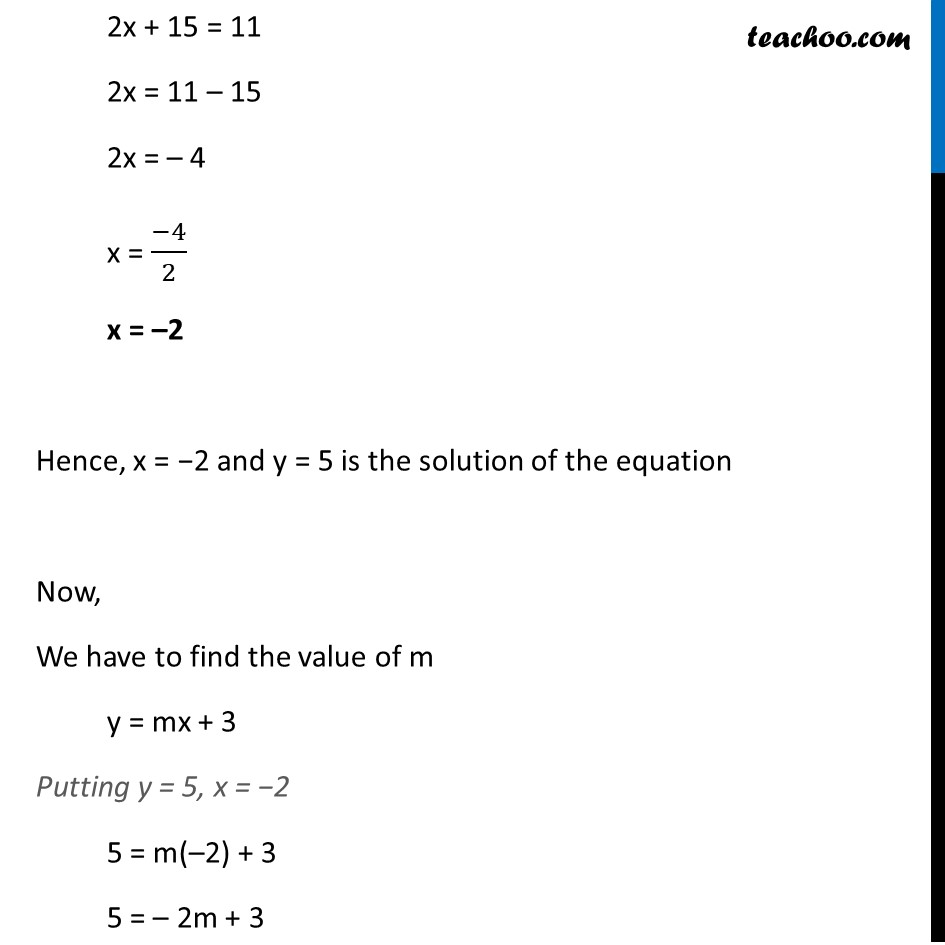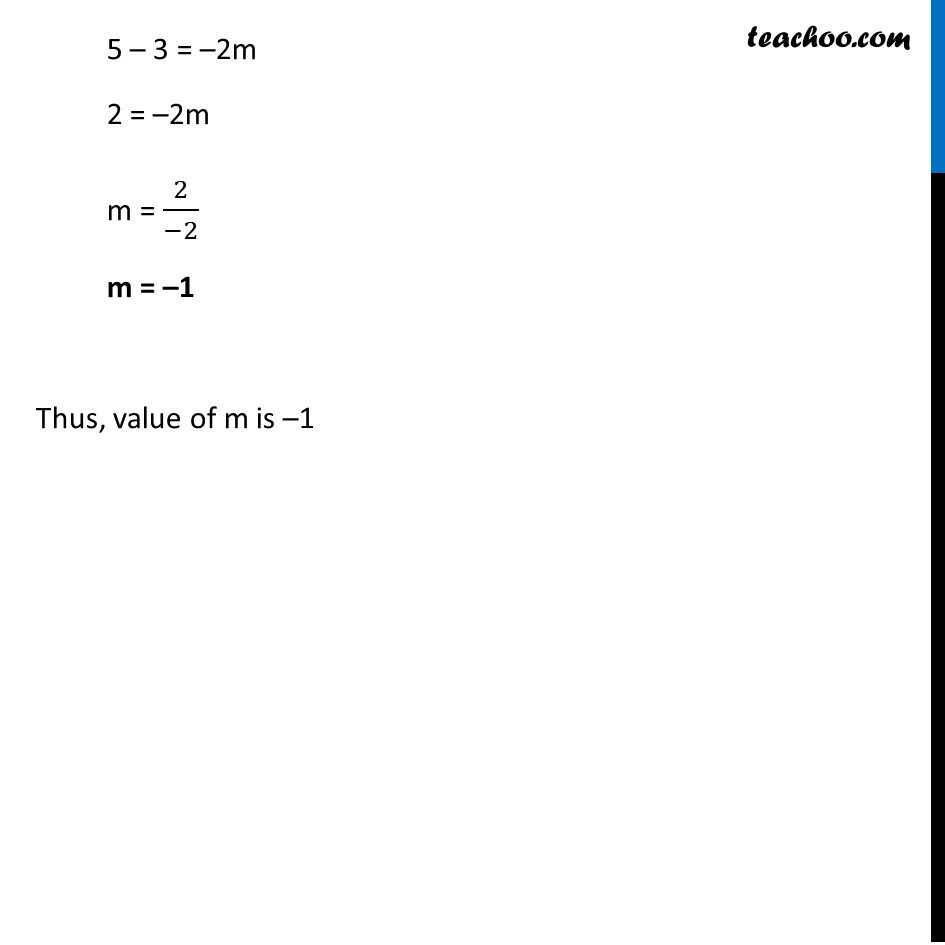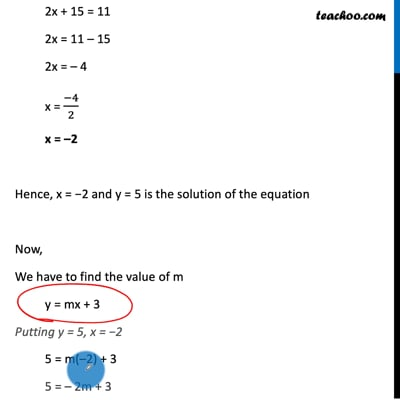This video is only available for Teachoo black users

Maths Crash Course - Live lectures + all videos + Real time Doubt solving!

### Transcript

Ex 3.3, 2 Solve 2x + 3y = 11 and 2x – 4y = – 24 and hence find the value of ‘m’ for which y = mx + 3. 2x + 3y = 11 2x – 4y = –24 From (1) 2x + 3y = 11 2x = 11 – 3y x = (𝟏𝟏 − 𝟑𝒚)/𝟐 Substituting value of x in (2) 2x – 4y = – 24 2 ((11 − 3𝑦)/2)−4𝑦 = – 24 11 – 3y – 4y = – 24 11 – 7y = – 24 –7y = – 24 – 11 –7y = – 35 y = (−35)/(−7) y = 5 Putting y = 5 in (1) 2x + 3y = 11 2x + 3(5) = 11 2x + 15 = 11 2x = 11 – 15 2x = – 4 x = (−4)/2 x = –2 Hence, x = −2 and y = 5 is the solution of the equation Now, We have to find the value of m y = mx + 3 Putting y = 5, x = −2 5 = m(–2) + 3 5 = – 2m + 3 5 – 3 = –2m 2 = –2m m = 2/(−2) m = –1 Thus, value of m is –1